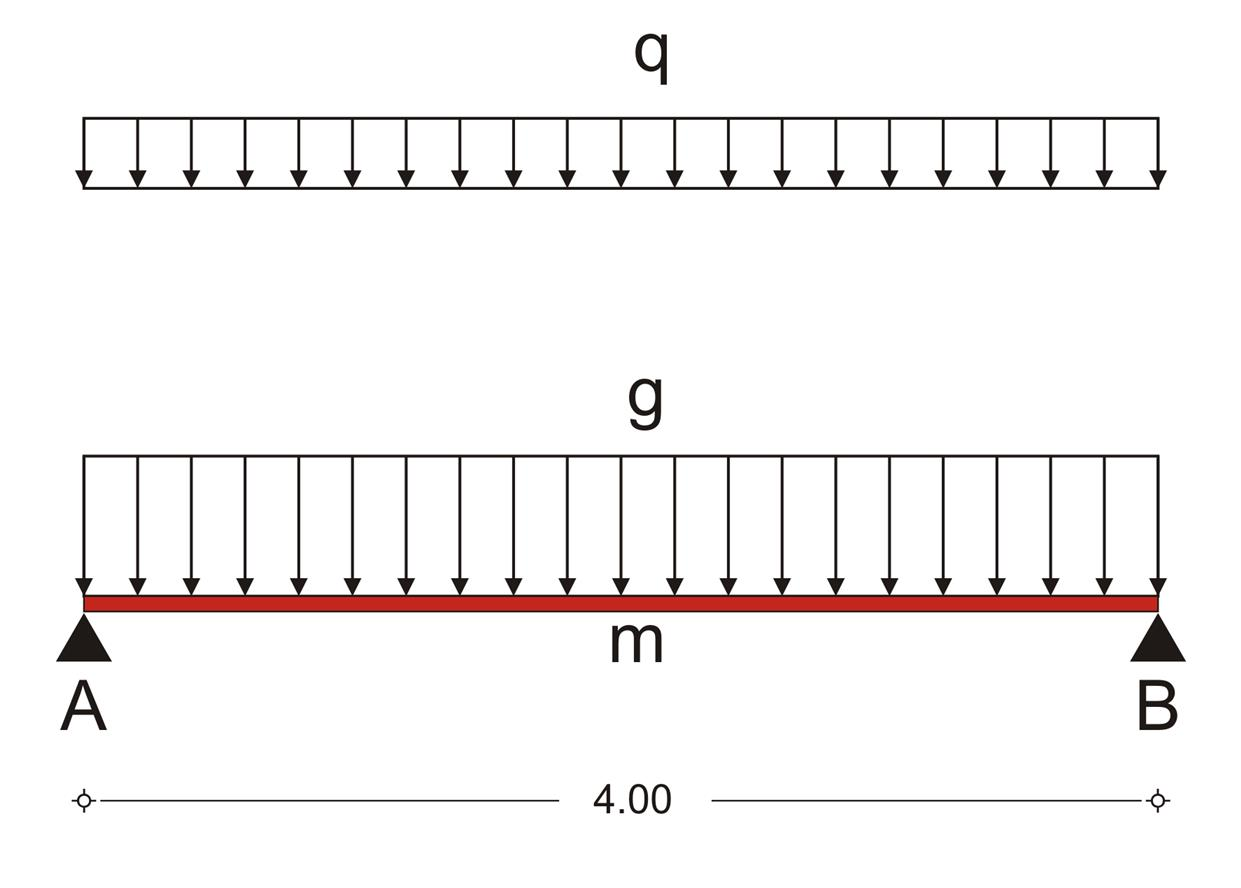« Frame model Analysis of frames » Analysis of slab The slabs of reinforced concrete structures can be modelled as bars with width 1.0 m hinged on beams [*]NoteSlab together with beams and columns behave as one structure in which each member affects and is affected by the others. In case of reinforced concrete structures, in §3.3.4 is indicated and in Annex Α is proved, that the separate analysis of the slab, hingedly supported on beams, gives the most reliable results.Figure 3.3.2: The equivalent bar, hinged at both ends, of the slab strip Figure 3.3.2: The equivalent bar, hinged at both ends, of the slab strip In this example, slab s1 is hinged at both ends with width h=170 mm, and span l=4.00 m. Τhe design stress resultants, result from the slab's self-weight and covering loads and a live load q=5.00 kN/m2. The analysis models the slab as a strip of width 1.00 m. The self-weight of concrete is 25.0 kN/m3 therefore: Self-weight: go=0.17mx1.00mx25.00kN/m3 =4.25 kN/m Covering: ge=1.00mx1.00kN/m2 =1.00 kN/m Total dead loads: g =5.25 kN/m Total live loads: q=1.00mx5.00kN/m2 =5.00 kN/m The design combination for the ultimate limit state derives from the design load psd=γg·g+γq·q which in this case is: psd=1.35g+1.50q=1.35x5.25+1.50x5.0=14.59 kN/m, therefore, the stress resultants used for the slab design, derive from the expressions: V Ad =-V Bd =14.59x(4.00/2)=29.18 kN M md =14.59x(4.002/8)=29.18 kNm « Frame model Analysis of frames »